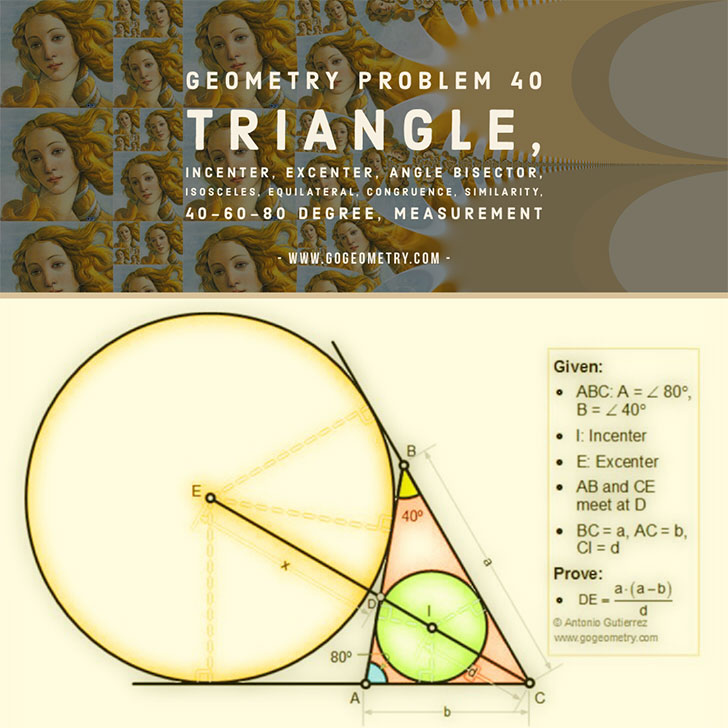Geometry Problem 40

Triangle, Incenter, Excenter, 40, 60, 80 Degrees

Proposition

In a triangle ABC, the angles A and B measure 80 and 40 respectively, I is the incenter and E the excenter. AB and CE meet at D. If BC = a, AC = b and CI = d, prove that: $$DE= \dfrac{a \cdot (a-b)}{d}$$.Poster of problem 40